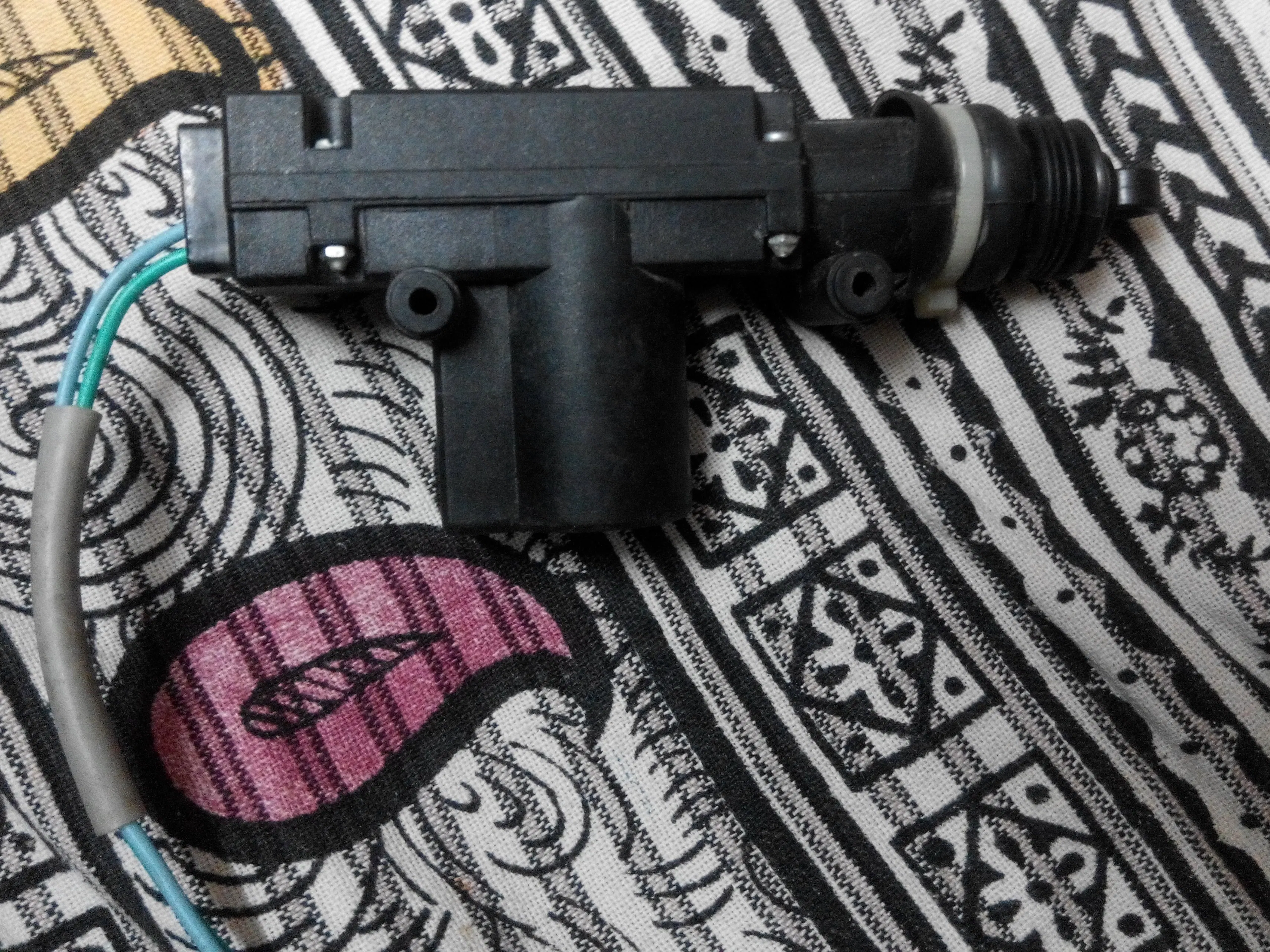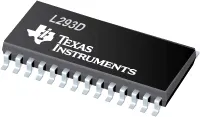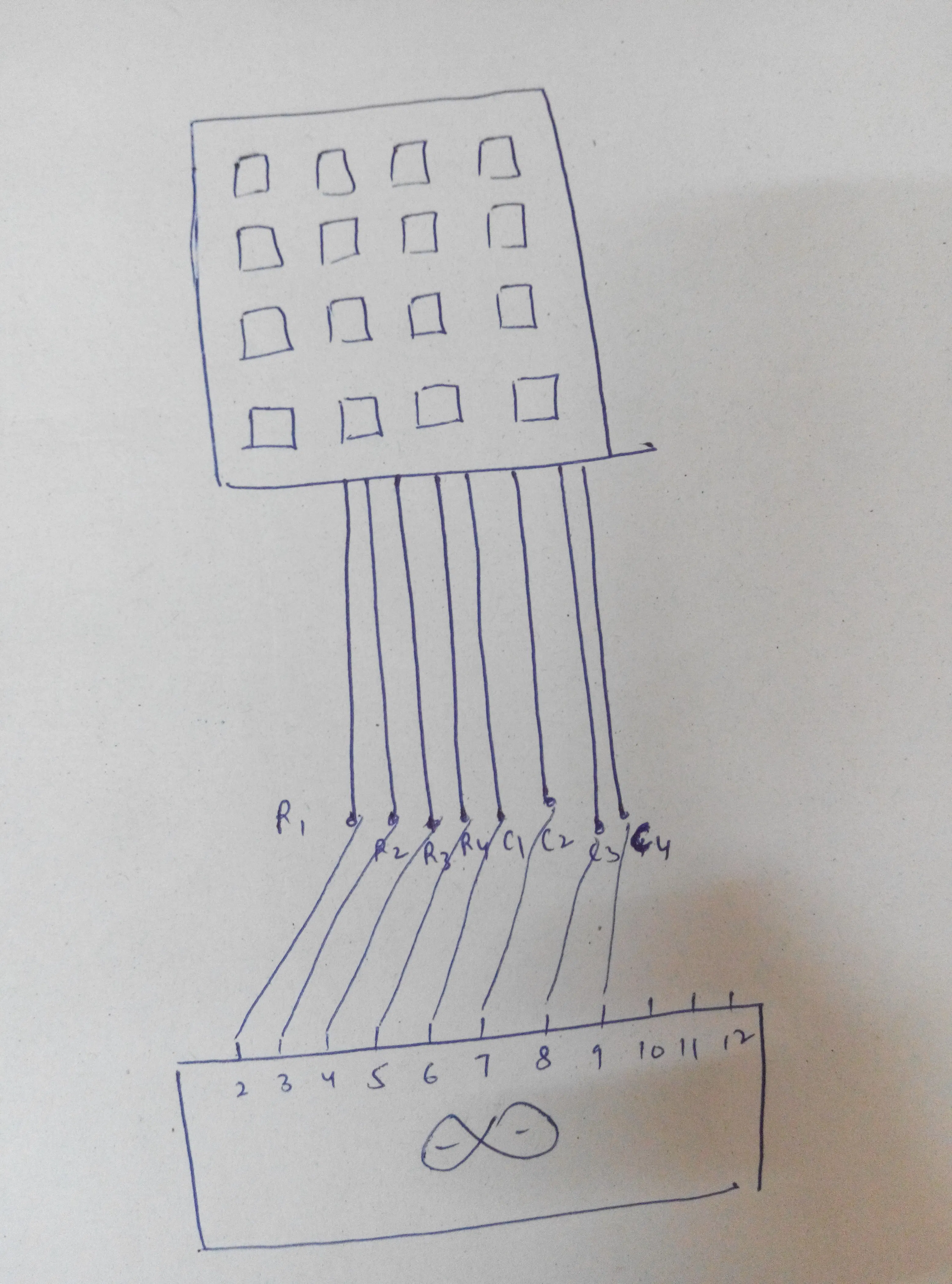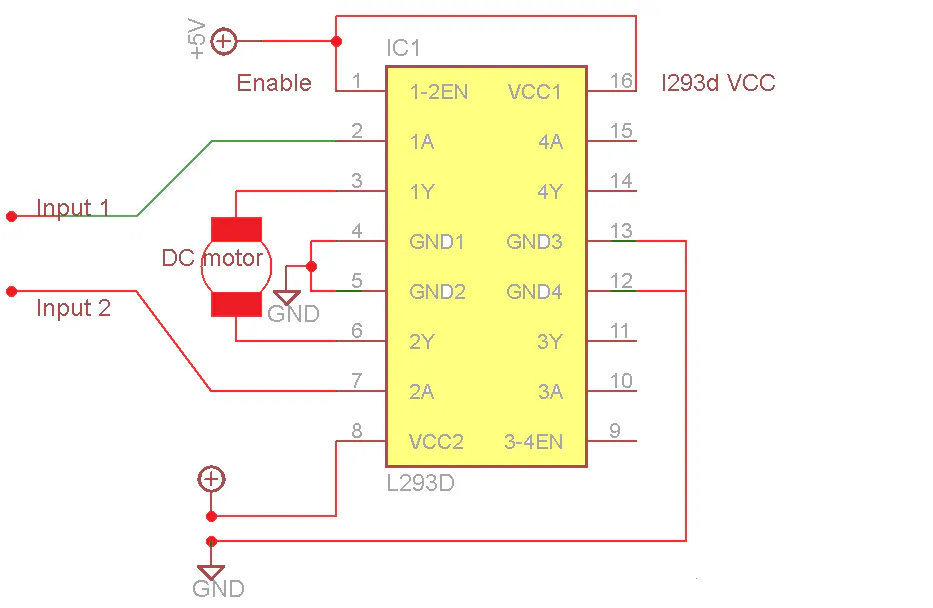BeginnerFull instructions provided1.5 hours17,930## Things used in this project

### Hardware componentsArduino UNO & Genuino UNO
×1
×1Texas Instruments Dual H-Bridge motor drivers L293D
×1
 linear actuator
×1

## Schematics### l293d connection

Connect actuator where the motor is connected.
Connect pin 8 to 12v### Video 1

pressed key displayed on the screen

### Video 2

led glows when the password is correct

### Video 3

The actuator is pulled outside in normal case (i.e. the door is locked).
When the password is correct , it is pulled inside and the door opens

## Code

### code 1

Arduino
Scanning
```int rows=4;
int columns=4;
char nfunc(void);
#define c3 9
#define c2 8
#define c1 7
#define c0 6
#define r3 5
#define r2 4
#define r1 3
#define r0 2
void setup() {
Serial.begin(9600);
pinMode(c0,INPUT_PULLUP);
pinMode(c1,INPUT_PULLUP);
pinMode(c2,INPUT_PULLUP);
pinMode(c3,INPUT_PULLUP);
pinMode(r0,OUTPUT);
pinMode(r1,OUTPUT);
pinMode(r2,OUTPUT);
pinMode(r3,OUTPUT);
pinMode(11,OUTPUT);
pinMode(12,OUTPUT);

}
{digitalWrite(r0,LOW);
digitalWrite(r1,HIGH);
digitalWrite(r2,HIGH);
digitalWrite(r3,HIGH);
return '1';}
return '2';}
return '3';}
return 'A';}

digitalWrite(r0,HIGH);
digitalWrite(r1,LOW);
digitalWrite(r2,HIGH);
digitalWrite(r3,HIGH);
return '4';}
return '5';}
return '6';}
return 'B';}

digitalWrite(r0,HIGH);
digitalWrite(r1,HIGH);
digitalWrite(r2,LOW);
digitalWrite(r3,HIGH);
return '7';}
return '8';}
return '9';}
return 'C';}

digitalWrite(r0,HIGH);
digitalWrite(r1,HIGH);
digitalWrite(r2,HIGH);
digitalWrite(r3,LOW);
return '*';}
return '0';}
return '#';}
return 'D';}
return 'o';
}

char nfunc(void)
{
char key='o';
while(key=='o')
return key;
}

void loop()
{
char key1= nfunc();

if(key1){
Serial.print(key1);

}

}
```

### code 2

Arduino
```int rows=4;
int columns=4;
int count=0;
char nfunc(void);
#define c3 9
#define c2 8
#define c1 7
#define c0 6
#define r3 5
#define r2 4
#define r1 3
#define r0 2
char typed;

void setup() {
Serial.begin(9600);
pinMode(c0,INPUT_PULLUP);
pinMode(c1,INPUT_PULLUP);
pinMode(c2,INPUT_PULLUP);
pinMode(c3,INPUT_PULLUP);
pinMode(r0,OUTPUT);
pinMode(r1,OUTPUT);
pinMode(r2,OUTPUT);
pinMode(r3,OUTPUT);
pinMode(11,OUTPUT);
pinMode(12,OUTPUT);

}
{digitalWrite(r0,LOW);
digitalWrite(r1,HIGH);
digitalWrite(r2,HIGH);
digitalWrite(r3,HIGH);
return '1';}
return '2';}
return '3';}
return 'A';}

digitalWrite(r0,HIGH);
digitalWrite(r1,LOW);
digitalWrite(r2,HIGH);
digitalWrite(r3,HIGH);
return '4';}
return '5';}
return '6';}
return 'B';}

digitalWrite(r0,HIGH);
digitalWrite(r1,HIGH);
digitalWrite(r2,LOW);
digitalWrite(r3,HIGH);
return '7';}
return '8';}
return '9';}
return 'C';}

digitalWrite(r0,HIGH);
digitalWrite(r1,HIGH);
digitalWrite(r2,HIGH);
digitalWrite(r3,LOW);
return '*';}
return '0';}
return '#';}
return 'D';}
return 'o';
}

char nfunc(void)
{
char key='o';
while(key=='o')
return key;
}

void loop()
{
char key1= nfunc();

if(key1){
typed[count]=key1;
count++;
Serial.print(key1);

}
if(count==4){
{
Serial.println(" correct");
digitalWrite(11,HIGH);
digitalWrite(12,LOW);
delay(250);
digitalWrite(11,LOW);
}
else{
Serial.println(" intruder");
}
count=0;
}}
```

## Credits

### Rishabh

4 projects • 26 followers
I am an ECE engineer with embedded systems , circuit designing and Hardware engineering as my areas of interest. plz check my projects out